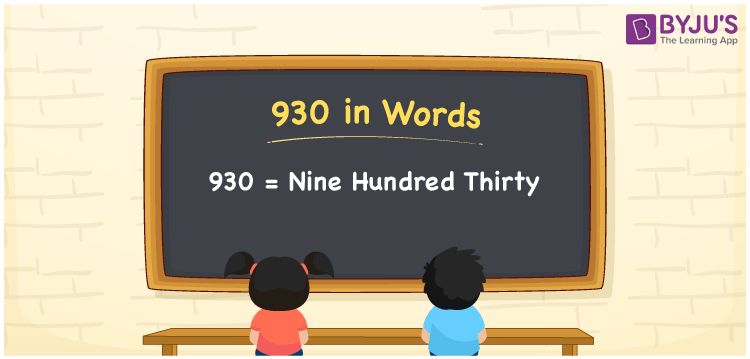# 930 in Words

In English, the number 930 is written as “Nine Hundred Thirty”’. In Mathematics, the number 930 is a natural number that comes after the number 929 but before the number 931. Using place values, such as ones, tens and hundreds, the number can be written in words. In this article, let us discuss how to write the number 930 in words and its procedure in detail.

 930 in Words: Nine Hundred Thirty. Nine Hundred Thirty in Numerical Form: 930.

## 930 in English Words## How to Write 930 in Words?

Using the below place value table of 930, the number 930 in words can be written as follows:

 Hundreds Tens Ones 9 3 0

The expanded form of 930 is as follows:

= 9 × Hundred + 3 × Ten + 0 × One

= 9 × 100 + 3 × 10 + 0 × 1

= 900 + 30

= 930

= Nine hundred thirty

Hence, 930 in words is nine hundred thirty.

930 in words – Nine hundred thirty

Is 930 an odd number? – No

Is 930 an even number? – Yes

Is 930 a perfect square number? – No

Is 930 a perfect cube number? – No

Is 930 a prime number? – No

Is 930 a composite number? – Yes

## Frequently Asked Questions on 930 in Words

### How to write 930 in English words?

930 in words is nine hundred thirty.

### Simplify 900 + 30, and express it in words.

Simplifying 900 + 30, we get 930. Hence, 930 in words is nine hundred thirty.

### Is 930 an even number?

Yes, 930 is an even number.